# Gravitation NCERT solutions

Question 8.1
(a) You can shield a charge from electrical forces by putting it inside a hollow conductor. Can you shield a body from the gravitational influence of nearby matter by putting it inside a hollow sphere or by some other means?
(b) An astronaut inside a small spaceship orbiting around the Earth cannot detect gravity. If the space station orbiting around the Earth has a large size, can he hope to detect gravity?
(c) If you compare the gravitational force on the Earth due to the Sun to that due to the Moon you would find that the Sun's pull is greater than the Moon's pull. (You can check this yourself using the data available in the succeeding exercises). However, the tidal effect of the Moon's pull is greater than the tidal effect of Sun. Why?
Solution
(a) No . It is not possible to body to shield from the gravitational influence of nearby matter. The reason is that gravitational forces unlike electrical forces are independent of the nature of the material medium in which bodies are placed.
(b) Yes. If the size of the spaceship orbiting around the earth is large, then the gravitational effect of spaceship may become measurable.
(c) The tidal effect depends inversely upon the cube of the distance whereas the gravitational force varies inversely as the square of the distance. As the moon is closer to the earth then sun , so its tidal effect is greater than that that of sun.

Question 8.2
Choose the correct alternative:
(a) Acceleration due to gravity increases/ decreases with increasing altitude.
(b) Acceleration due to gravity increases/ decreases with increasing depth (assume the Earth to be a sphere of uniform density).
(c) Acceleration due to gravity is independent of the mass of the Earth/mass of the body.
(d) The formula $-GMm\left( \frac{1}{{{r}_{2}}}-\frac{1}{{{r}_{1}}} \right)$ is more/ less accurate than the formula $mg({{r}_{2}}-{{r}_{1}})$ for the difference of potential energy between two points $r_2$ and $r_1$ distance away from the center of the Earth.
Solution
(a) Acceleration due to gravity at an altitude $h$ is given by the formula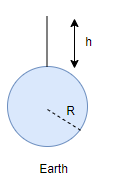$g_h=g_0\left( 1-\frac{2h}{R} \right)$
So acceleration due to gravity decreases with increasing altitude.
(b) Acceleration due to gravity at depth $d$ is given by the formula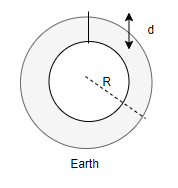$g_d=\left( 1-\frac{d}{R} \right)$ .
Thus acceleration due to gravity decreases with increasing depth.
(c) Acceleration due to gravity is given as
$g=\frac{GM}{R^2}$, where $M$ is the mass of the earth.
It is clear that the acceleration due to gravity is independent of the mass $m$ of the body.
(d) The formula $-GMm\left( \frac{1}{{{r}_{2}}}-\frac{1}{{{r}_{1}}} \right)$ is more accurate than the formula $mg({{r}_{2}}-{{r}_{1}})$ as value of g varies from place to place.

Question 8.3
Suppose there existed a planet that went around the sun twice as fast as the earth. What would be its orbital size as compared to that of the earth?
Solution
Let $T_1$ and $T_2$ be the period of revolution of earth and planet in question.Since planet goes around sun twice as fast as earth.
$T_2=\frac{1}{2}T_1$
Orbital size of earth = $r_e = 1 \ AU$
and orbital size of the planet = $r_p = ?$
Now, from Kepler's third law of planetary motion,
$\frac{T_{p}^{2}}{T_{e}^{2}}=\frac{r_{p}^{3}}{r_{e}^{3}}$
${{r}_{p}}={{\left( \frac{{{T}_{p}}}{{{T}_{e}}} \right)}^{{}^{2}/{}_{3}}}{{r}_{e}}={{\left( \frac{\frac{1}{2}{{T}_{e}}}{{{T}_{e}}} \right)}^{{}^{2}/{}_{3}}}\times 1AU$
${{r}_{p}}={{(0.5)}^{{}^{2}/{}_{3}}}AU=0.63AU$

Question 8.4
Io, one of the satellites of Jupiter, has an orbital period of 1.769 days and the radius of the orbit is $4.22 \times 10^8 \ m$. Show that mass of Jupiter is about one-thousandth times that of the sun. (Take 1 year = 365.25 mean solar day)
Solution
Given
For the satellite of Jupiter, $T_J = 1.769 \ days = 1.769 \times 24 \times 60 \times 60 \ s$
Orbital radius of Jupiter satellite, $R_J = 4.22 \times 10^8 \ m$.
Now we know from Keplers law
$T_J^2 = \frac {4 \pi ^2 R_J^2}{G M_J}$
Therefore Mass of Jupiter will be given by
${{M}_{J}}=\frac{4{{\pi }^{2}}R_{J}^{3}}{GT_{J}^{2}}$
$\Rightarrow {{M}_{J}}=\frac{4{{\pi }^{2}}\times {{(4.22\times {{10}^{8}})}^{3}}}{G{{(1.769\times 24\times 60\times 60)}^{2}}}$
Orbital period of earth around the sun
$T=1 \ year = 365.25 \times 24 \times 60 \times 60 \ seconds$
$R=1.469 \times 10{11}$ m
Now we know from Keplers law
$T^2 = \frac {4 \pi ^2 R^2}{G M_s}$
Therefore Mass of the sun
${{M}_{s}}=\frac{4{{\pi }^{2}}{{R}^{2}}}{G{{T}^{2}}}=\frac{4{{\pi }^{2}}}{G}\frac{{{(1.496\times {{10}^{11}})}^{3}}}{{{(365.25\times 24\times 60\times 60)}^{2}}}$
Comparing Mass of Jupiter and Mass of Sun
\begin{align} & \frac{{{M}_{J}}}{{{M}_{S}}}=\frac{4{{\pi }^{2}}\times {{(4.22\times {{10}^{8}})}^{3}}}{G{{(1.769\times 24\times 60\times 60)}^{2}}}\cdot \frac{G}{4{{\pi }^{2}}}\frac{{{(365.25\times 24\times 60\times 60)}^{2}}}{{{(1.496\times {{10}^{11}})}^{3}}} \\ & \frac{{{M}_{J}}}{{{M}_{S}}}=\frac{1}{1046}\approx \frac{1}{1000} \\ \end{align} Hence mass of Jupiter is about one thousandth that of sun.

Question 8.5
Let us consider that our galaxy consists of $2.5 \times 10^{11}$ stars each of one solar mass. How long will this star at a distance of 50,000 lightyear from the galactic centre take to complete one revolution? Take the diameter of the Milky way to be $10^5$ lightyear and $G = 6.67 \times 10^{-11} \ Nm^2/kg^2$
Given 1 Solar Mass=$2 \times 10^{30} \ Kg$
Solution
Mass of galaxy (M)=$2.5 \times 10^{11} \ \text{solar masses} = 2.5 \times 10^{11} \times 2 \times 10^{30} \ Kg = 5 \times 10^{41} \ Kg$
Orbital radius of the star (R) = $50000 \ ly = 50000 \times 9.46 \times 10^{15}= 4.73 \times {{10}^{20}}$ m
Now from Kepler's Law
$T^2= \frac{{4{\pi ^2}{R^2}}}{{GM}}$
Putting values given in above equation we get
${{T}^{2}}=\frac{4\times 9.87\times {{(4.73\times {{10}^{20}})}^{3}}}{6.67\times {{10}^{-11}}\times 5\times {{10}^{41}}}=1.25275\times {{10}^{32}}s$
$\therefore T=1.12\times {{10}^{16}}s=\frac{1.12\times {{10}^{16}}}{365.25\times 24\times 60\times 60}years=3.54\times {{10}^{8}}years$

Question 8.6
Choose the correct alternative:
(a) If the zero of potential energy is at infinity, the total energy of an orbiting satellite is negative of its kinetic/potential energy.
(b) The energy required to launch an orbiting satellite out of Earth's gravitational influence is more/less than the energy required to project a stationary object at the same height (as the satellite) out of Earth's influence.
Solution
(a) If the zero of potential energy is at infinity, the total energy of an orbiting satellite is negative of its kinetic energy.
(b) The energy required to launch an orbiting satellite out of Earth's gravitational influence is less than the energy required to project a stationary object at the same height (as the satellite) out of earth's influence.

Question 8.7
Does the escape speed of a body from the Earth depend on
(a) the mass of the body,
(b) the location from where it is projected,
(c) the direction of projection,
(d) the height of the location from where the body is launched?
Solution
The escape velocity is given by the formula
${{v}_{e}}=\sqrt{\frac{2GM}{r}}$
It can be easily observed from the formula
(a) It is independent of the mass of the body.
(b) It does not depend on the location from where a body is projected.
(c) It does not depend on the direction of projection of a body.
(d) The escape velocity depends upon the gravitational potential at the point from which it is projected and that depends on height also . So Escape velocity depends upon the height of the location from where the body is projected .

Question 8.8
A comet orbits the Sun in a highly elliptical orbit. Does the comet have a constant
(a) linear speed
(b) angular speed
(c) angular momentum
(d) kinetic energy
(e) potential energy
(f) total energy throughout its orbit?
Neglect any mass loss of the comet when it comes very close to the Sun.
Solution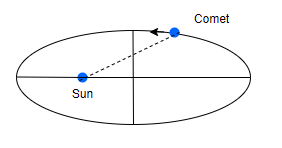(a) The linear speed of the comet keeps on changing in accordance with Kepler's second law of planetary motion. When comet is near the sun, its speed is higher. When the comet is far away from the sun, its speed is very less.
(b) The angular speed also varies.
(c) Comet has constant angular momentum
(d) Kinetic energy does not remain constant as Linear speed varies
(e) Potential energy varies along the path as the distance of the comet from sun changes continuously.
(f) Total energy throughout the orbit remains constant.

Question 8.9
Which of the following symptoms is likely to afflict an astronaut in space
(a) swollen feet
(b) swollen face
(d) orientation problem.
Solution
(a) No. We know that the legs carry the weight of the body in the normal position due to gravity pull. The astronaut in space is in weightlessness state. Hence, swollen feet may not affect his working.
(b) Yes.An astronaut may develop swollen face because of weightlessness state. Swollen face may affect to great extent the seeing/hearing/ smelling/ eating capabilities of the astronaut in space.
(c) Yes.Headache is due to mental strain. It will persist whether a person is an astronaut in space or he is on earth. It means headache will have the same effect on the astronaut in space as on a person on earth.
(d) Yes. Space also has orientation. We also have the frames of reference in space. Hence, orientation problem will affect the astronaut in space.

Question 8.10
In the following two exercises, choose the correct answer from among the given ones. The gravitational intensity at the centre of a hemispherical shell of uniform mass density has the direction indicated by the arrow (see Fig.) (i) a, (ii) b, (iii) c, (iv) 0.Solution• To solve this problem let us consider a complete spherical shell. We know that the gravitational potential (V) is constant at all points inside a spherical shell. Therefore, gravitational potential gradient $\left( -\frac{dV}{dr} \right)$ at all points inside a spherical shell is zero.
• Since gravitational intensity is equal to the negative of gravitational potential gradient, hence the gravitational intensity is zero at all points inside a hollow spherical shell. This indicates that the gravitational force acting on a particle at any point inside spherical shell , will be symmetrically placed.
• If we remove the upper half of the complete spherical shell, we get the hemispherical shell. Since the gravitational intensity at Center C is zero, the direction of gravitational intensity must be downwards as shown by arrow c. Hence option (iii) is correct.

Question 8.11
For the above problem, the direction of the gravitational intensity at an arbitrary point P is indicated by the arrow (i) d, (ii), e, (iii) f (iv) g.
Solution
Using the explanation given in the solution of the previous problem, the direction of the gravitational field intensity at P will be along e. So, option (ii) is correct.

Question 8.12
A rocket is fired from the earth towards the sun. At what point on its path is the gravitational force on the rocket zero?
Mass of sun = $2 \times 10^{30} \ kg$, Mass of earth = $6 \times 10^{24} \ kg$.
Neglect the effect of the other planets. Orbital radius of the earth = $1.5 \times 10^{11} \ m$
Solution
Given
$M_s=2 \times 10^{30} \ kg$
$M_e=6 \times 10^{24} \ kg$
$r=1.5 \times 10^{11} \ m$
Let m be the mass of the rocket. Let at a distance x from the earth, the gravitational force on the rocket due to sun and the earth are equal and opposite.
Therefore distance of the rocket from the sun $=r-x$
Gravitational pull of the earth on the rocket = gravitational pull of the sun on the rocket.
$\frac{G{{M}_{S}}m}{{{(r-x)}^{2}}}=\frac{G{{M}_{E}}m}{{{x}^{2}}}\Rightarrow \frac{{{(r-x)}^{2}}}{{{x}^{2}}}=\frac{{{M}_{S}}}{{{M}_{E}}}$
$\frac{r-x}{x}=\sqrt{\frac{{{M}_{S}}}{{{M}_{E}}}}\Rightarrow \sqrt{\frac{2\times {{10}^{30}}}{6\times {{10}^{24}}}}=\frac{{{10}^{3}}}{\sqrt{3}}$
$\frac{r}{x}-1=\frac{{{10}^{3}}}{\sqrt{3}}$
Solving it for x we get
$x=\frac{\sqrt{3}r}{{{10}^{3}}+\sqrt{3}}=\frac{1.732\times {{10}^{11}}\times 1.5}{{{10}^{3}}+1.732}=2.59\times {{10}^{8}}m$

Question 8.13
How will you 'weigh the sun', that is estimate its mass? The mean orbital radius of the earth around the sun is $1.5 \times 10^8$ km.
Solution
The mean orbital radius of the Earth around the Sun
$R = 1.5 \times 10^8 \ km = 1.5 \times 10^{11}$ m
Time period, $T = 365.25 \times 24 \times 60 \times 60 s$
Let the mass of the Sun be $M$ and that of Earth be $m$. According to law of gravitation
$F=G\frac{Mm}{{{R}^{2}}}$
Centripetal force,
$F=\frac{m{{v}^{2}}}{R}=mR{{\omega }^{2}}$
Equating both these forces
$G\frac{Mm}{{{R}^{2}}}=mR{{\omega }^{2}}$
since $\omega =\frac{2\pi }{T}$
$\therefore M=\frac{4{{\pi }^{2}}{{R}^{3}}}{G{{T}^{2}}}$
$M=\frac{4\times {{(3.14)}^{2}}\times {{(1.5\times {{10}^{11}})}^{3}}}{6.67\times {{10}^{-11}}\times {{(365.25\times 24\times 60\times 60)}^{2}}}$
after calculating we get
$M=2.0\times {{10}^{30}}Kg$

Question 8.14
A Saturn year is 29.5 times the earth year. How far is the Saturn from the Sun if the Earth is $1.5 \times 10^8$ km away from the sun?
Solution
$T_s = 29.5 T_e$, $R_e = 1.5 \times 10^8$ km
From Kepler's 3rd law,
$\frac{T_{s}^{2}}{T_{e}^{2}}=\frac{R_{s}^{3}}{R_{e}^{3}}$
Therefore,
\begin{align} & {{R}_{s}}={{R}_{e}}{{\left( \frac{{{T}_{s}}}{{{T}_{e}}} \right)}^{{}^{2}/{}_{3}}}=1.5\times {{10}^{8}}{{\left( \frac{29.5{{T}_{e}}}{{{T}_{e}}} \right)}^{{}^{2}/{}_{3}}} \\ & {{R}_{s}}=1.43\times {{10}^{9}}Km \\ \end{align}

Question 8.15
A body weighs 63N on the surface of the earth. What is the gravitational force on it due to the earth at a height equal to half the radius of the earth?
Solution
Weight of the body = mg = 63N
height $h=\frac{R}{2}$
Accleration due to gravity at a height is given by
$g_h=g {{\left( \frac{R}{R+h} \right)}^{2}}$
$\frac{{{g}_{h}}}{g}={{\left( \frac{R}{R+h} \right)}^{2}}={{\left( \frac{R}{R+\frac{R}{2}} \right)}^{2}}={{\left( \frac{2}{3} \right)}^{2}}=\frac{4}{9}$
${{g}_{h}}=\frac{4}{9}g$
Therefore,
$m{{g}_{h}}=\frac{4}{9}mg=\frac{4}{9}\times 63=28N$

Question 8.16
Assuming the earth to be a sphere of a uniform mass density, how much would a body weigh half way down to the centre of earth if it weighed 250 N on the surface? [g on the surface of the earth = $9.8 \ m/s^2$]
Solution
Given
$\text{Weight}= mg=250 \ N$
$d=\frac{R}{2}$
Acceleration due to gravity at a depth $d$ below the surface of earth will be
${{g}_{d}}=g\left( 1-\frac{d}{R} \right)$
Substituting the values
${{g}_{d}}=g\left( 1-\frac{{\scriptstyle{}^{R}/{}_{2}}}{R} \right)=\frac{g}{2}$
Hence,
New weight = $m{{g}_{d}}=\frac{mg}{2}=\frac{250}{2}=125N$

Question 8.17
A rocket is fired vertically with a speed of 5 km/s from the earth's surface. How far from the earth does the rocket go before returning to the earth?
Mass of the earth = $6 \times 10^{24} \ kg$, Mean radius of the earth = $6.4 \times 10^6 \ m$, $G = 6.67 \times 10^{-11} \ Nm^2/kg^2$
Solution
Let, v = velocity of the rocket when it is fired from the earth.
h = height at which its velocity becomes zero.
Total energy of the rocket at the surface of the earth
$E_1 =K.E + P.E=\frac{1}{2}m{{v}^{2}}+\left( -\frac{GMm}{R} \right)$
At highest point
$K.E.=0$ and $P.E.=\left( -\frac{GMm}{R+h} \right)$
Therefore,
$E_2=\left( -\frac{GMm}{R+h} \right)$
Using the law of conservation of energy,
$E_1= E_2$
$\frac{1}{2}m{{v}^{2}}-\left( \frac{GMm}{R} \right)=-\left( \frac{GMm}{R+h} \right)$
$\frac{1}{2}{{v}^{2}}=GM\left( \frac{1}{R}-\frac{1}{R+h} \right)=GM\left( \frac{R+h-R}{R(R+h)} \right)$
Now we know that
$g=\frac {GM}{R^2}$
Therefore
$\frac{1}{2}{{v}^{2}}=\frac{g{{R}^{2}}h}{R(R+h)}$
$\frac{1}{2}{{v}^{2}}=\frac{gRh}{R+h}$
${{v}^{2}}(R+h)=2gRh$
on rearranging above equation we find
$h=\frac{{{v}^{2}}R}{2gR-{{v}^{2}}}$
Substituting all the values,we get
$h=\frac{{{(5\times {{10}^{3}})}^{2}}\times 6.4\times {{10}^{6}}}{(2\times 9.8\times 6.4\times {{10}^{6}})-{{(5\times {{10}^{3}})}^{2}}}=1.6\times {{10}^{6}}m$

Question 8.18
The escape velocity of a projectile on the surface of earth is 11.2 km/s. If a body is projected out with thrice of this speed, find the speed of the body far away from the earth. Ignore the presence of other planets and sun.
Solution
Given that escape velocity is $v_e = 11.2 km/s$,
velocity of projection, $v = 3v_e$
Let $m$ and $v_o$ be the mass and the velocity of the projectile far away from the earth then using law of conservation of energy,
$\frac{1}{2}m v_0^2=\frac{1}{2}m{{v}^{2}}-\frac{1}{2}mv_{e}^{2}$
${{v}_{0}}=\sqrt{{{v}^{2}}-v_{e}^{2}}=\sqrt{{{(3{{v}_{e}})}^{2}}-v_{e}^{2}}=\sqrt{8}{{v}_{e}}$
putting the values we get
${{v}_{0}}=2\sqrt{2}\times 11.2=31.68 \ Km/s$

Question 8.19
A satellite orbits the earth at a height of 400 km above the surface. How much energy must be expended to rocket the satellite out of the gravitational influence of the earth? Mass of the satellite is 200 kg, mass of the earth = $6 \times 10^24 \ kg$, radius of the earth = $6.4 \times 10^6 \ m$, $G = 6.67 \times 10^{-11} \ Nm^2/kg^2$
Solution
Total energy of the satellite in the orbit is
$E=K.E.+P.E.=\frac{1}{2}m{{v}^{2}}-\frac{GMm}{R+h}$
Now Orbital velocity is given by
$v=\sqrt{\frac{GM}{R+h}}$
Therefore, Total energy will be given by
$E=\frac{1}{2}m\frac{GM}{R+h}-\frac{GMm}{R+h}=-\frac{GMm}{2(R+h)}$
This the energy with which sataellite is bounded with earth
Energy expended to rocket the satellite out of the earth's gravitational field = - (T.E. of the satellite) =$\frac{GMm}{2(R+h)}$
Substituting the values, we get
$\text{Energy required} =\frac{6.67\times10^{-11} \times 6\times10^{24} \times 200}{2(6.4 \times 10^6 + 4 \times 10^5)}=5.9 \times 10^9 \ J$

Question 8.20
Two stars each of 1 solar mass (= $2 \times 10^{30} \ kg$) are approaching each other for a head on collision. When they are at a distance $10^9 \ km$, their speeds are negligible. What is the speed with which they collide? The radius of each star is $10^4 \ km$. assume the stars to remain undisturbed until they collide. Use the known value of G.
Solution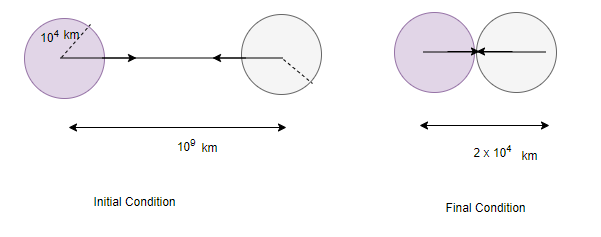Given
Mass of each star =$2 \times 10^{30} \ kg$
Initial distance between 2 stars = $10^9 \ km= 10^{12} \ m$
Initial P.E. of the system when they are $10^12 \ m$ apart
$U_i= - \frac {GM^2}{r} = - \frac {GM^2}{10^{12}}$
When the stars are just about to collide, distance between their centers= twice the radius of each star
Final potential energy of stars when they have just collide,
$U_f=-\frac{GM^2}{2R}=-\frac{GM^2}{2 \times 10^7}$
Let v be the speed of the stars,Then Total KE will be given as
$KE= \frac {1}{2} Mv^2 + \frac {1}{2} Mv^2=Mv^2$
Change in potential energies of stars
$U_i-U_f=\frac{-GM^2}{10^{12}}-\left(\frac{-GM^2}{2\times10^7}\right)$
$U_i-U_f=\frac{GM^2}{2\times10^7}-\frac{GM^2}{10^{12}} \sim eq\frac{GM^2}{2\times10^7}$
since $\frac{GM^2}{2\times10^7}\gg\frac{GM^2}{10^{12}}$
By conservation of energy
$Mv^2=\frac{GM^2}{2\times10^7}$
Substituting all the values
$v=2.58 \times 10^6 \ m/s$

Question 8.21
Two heavy spheres each of mass 100 kg and radius 0.10 m are placed 1.0 m apart on a horizontal table. What is the gravitational force and potential at the mid point of the line joining the centres of the spheres ? Is an object placed at that point in equilibrium? If so, is the equilibrium stable or unstable ?
Solution
The situation is shown in below figure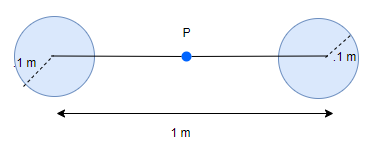Let r be the distance between them
Gravitational field at the mid point P of the line joining the two spheres is equal and opposite
$E= - \frac {GM}{{r/2}^2} + \frac {GM}{{r/2}^2}=0$
Gravitational Potential at the mid point P of the line joining the two spheres
$V= - \frac {GM}{r/2} - \frac {GM}{r/2}=-\frac {4GM}{r}$
Substituting the values
$V=-2.7 \times 10^{-8} \ J/kg$

Since Gravitational field at the mid point P of the line joining the two spheres is zero, object placed at that point will be in equilibrium.
But the equilibrium will be unstable as any change in position will change the effective force in that direction

Question 8.22
As you have learnt in the text, a geostationary satellite orbits the earth at a height of nearly 36,000 km from the surface of the earth. What is the potential due to earth's gravity at the site of this satellite ? (Take the potential energy at infinity to be zero).
Mass of the earth = $6.0 \times 10^{24} \ kg$, radius = 6400 km.
Solution
Gravitational potential at a height h above the surface of the earth is given by
$V= - \frac {GM}{R+h}$
Substituting all the values , we get
$V= -9.4 \times 10^6 \ J/kg$.

Question 8.23
A star 2.5 times the mass of the sun and collapsed to a size of 12 km rotates with a speed of 1.2 rev. per second. (Extremely compact stars of this kind are known as neutron stars. Certain stellar objects called pulsars belong to this category). Will an object placed on its equator remain stuck to its surface due to gravity ? (mass of the sun = $2 \times 10^{30} \ kg$).

Question 8.24
A spaceship is stationed on Mars. How much energy must be expended on the spaceship to launch it out of the solar system ? Mass of the space ship = 1000 kg; mass of the sun = $2 \times 10^{30} \ kg$ ; mass of mars = $6.4 \times 10^{23} \ kg$ ; radius of mars = 3395 km; radius of the orbit of mars = $2.28 \times 10^8 \ km$; $G = 6.67 \times 10^{-11} \ Nm^2 kg^{-2}$.

Question 8.25
A rocket is fired 'vertically' from the surface of mars with a speed of 2 km/s. If 20% of its initial energy is lost due to martian atmospheric resistance, how far will the rocket go from the surface of mars before returning to it ? Mass of mars = $6.4 \times 10^{23} \ kg$; radius of mars = 3395 km; $G = 6.67 \times 10^{-11} \ Nm^2 kg^{-2}$.